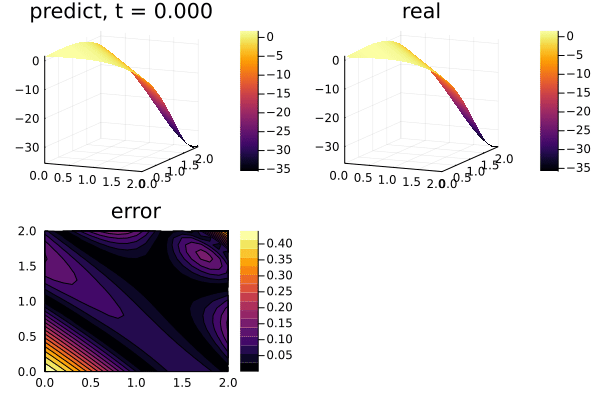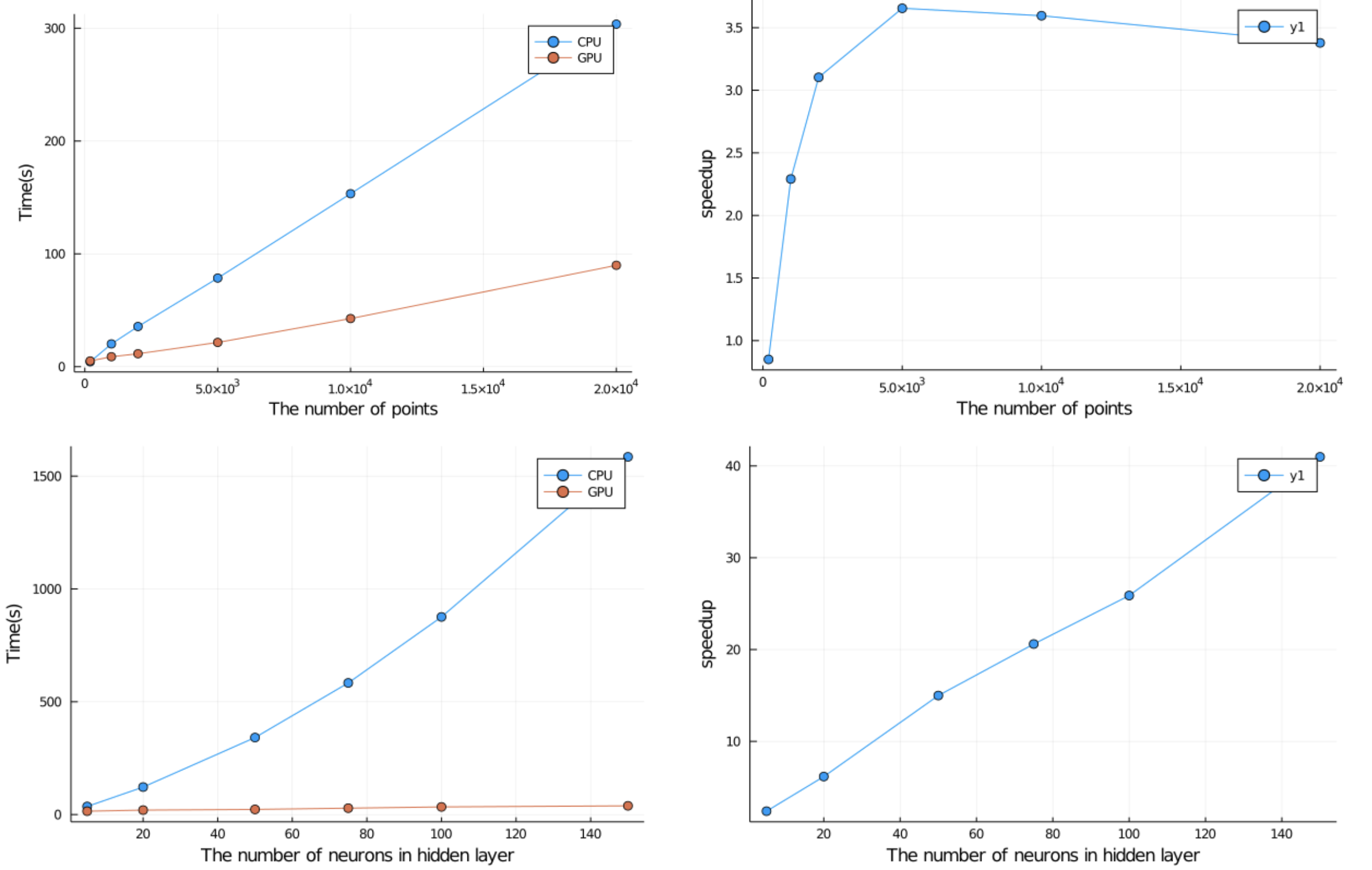# Using GPUs to train Physics-Informed Neural Networks (PINNs)

the 2-dimensional PDE:

$$$∂_t u(x, y, t) = ∂^2_x u(x, y, t) + ∂^2_y u(x, y, t) \, ,$$$

with the initial and boundary conditions:

\begin{align*} u(x, y, 0) &= e^{x+y} \cos(x + y) \, ,\\ u(0, y, t) &= e^{y} \cos(y + 4t) \, ,\\ u(2, y, t) &= e^{2+y} \cos(2 + y + 4t) \, ,\\ u(x, 0, t) &= e^{x} \cos(x + 4t) \, ,\\ u(x, 2, t) &= e^{x+2} \cos(x + 2 + 4t) \, , \end{align*}

on the space and time domain:

$$$x \in [0, 2] \, ,\ y \in [0, 2] \, , \ t \in [0, 2] \, ,$$$

with physics-informed neural networks. The only major difference from the CPU case is that we must ensure that our initial parameters for the neural network are on the GPU. If that is done, then the internal computations will all take place on the GPU. This is done by using the gpu function on the Flux.Chain, like:

using CUDA
chain = Chain(Dense(3,inner,Flux.σ),
Dense(inner,inner,Flux.σ),
Dense(inner,inner,Flux.σ),
Dense(inner,inner,Flux.σ),
Dense(inner,1)) |> gpu

In total, this looks like:

using NeuralPDE, Flux, CUDA
using Optimization, OptimizationOptimJL, OptimizationOptimsiers
import ModelingToolkit: Interval

@parameters t x y
@variables u(..)
Dxx = Differential(x)^2
Dyy = Differential(y)^2
Dt = Differential(t)
t_min= 0.
t_max = 2.0
x_min = 0.
x_max = 2.
y_min = 0.
y_max = 2.

# 2D PDE
eq  = Dt(u(t,x,y)) ~ Dxx(u(t,x,y)) + Dyy(u(t,x,y))

analytic_sol_func(t,x,y) = exp(x+y)*cos(x+y+4t)
# Initial and boundary conditions
bcs = [u(t_min,x,y) ~ analytic_sol_func(t_min,x,y),
u(t,x_min,y) ~ analytic_sol_func(t,x_min,y),
u(t,x_max,y) ~ analytic_sol_func(t,x_max,y),
u(t,x,y_min) ~ analytic_sol_func(t,x,y_min),
u(t,x,y_max) ~ analytic_sol_func(t,x,y_max)]

# Space and time domains
domains = [t ∈ Interval(t_min,t_max),
x ∈ Interval(x_min,x_max),
y ∈ Interval(y_min,y_max)]

# Neural network
inner = 25
chain = Chain(Dense(3,inner,Flux.σ),
Dense(inner,inner,Flux.σ),
Dense(inner,inner,Flux.σ),
Dense(inner,inner,Flux.σ),
Dense(inner,1)) |> gpu

strategy = GridTraining(0.05)
discretization = PhysicsInformedNN(chain,
strategy)

@named pde_system = PDESystem(eq,bcs,domains,[t,x,y],[u(t, x, y)])
prob = discretize(pde_system,discretization)
symprob = symbolic_discretize(pde_system,discretization)

callback = function (p,l)
println("Current loss is: $l") return false end res = Optimization.solve(prob,Adam(0.01);callback = callback,maxiters=2500) We then use the remake function allows to rebuild the PDE problem to start a new optimization at the optimized parameters, and continue with a lower learning rate: prob = remake(prob,u0=res.u) res = Optimization.solve(prob,Adam(0.001);callback = callback,maxiters=2500) Finally we inspect the solution: phi = discretization.phi ts,xs,ys = [infimum(d.domain):0.1:supremum(d.domain) for d in domains] u_real = [analytic_sol_func(t,x,y) for t in ts for x in xs for y in ys] u_predict = [first(Array(phi([t, x, y], res.u))) for t in ts for x in xs for y in ys] using Plots using Printf function plot_(res) # Animate anim = @animate for (i, t) in enumerate(0:0.05:t_max) @info "Animating frame$i..."
u_real = reshape([analytic_sol_func(t,x,y) for x in xs for y in ys], (length(xs),length(ys)))
u_predict = reshape([Array(phi([t, x, y], res.u)) for x in xs for y in ys], length(xs), length(ys))
u_error = abs.(u_predict .- u_real)
title = @sprintf("predict, t = %.3f", t)
p1 = plot(xs, ys, u_predict,st=:surface, label="", title=title)
title = @sprintf("real")
p2 = plot(xs, ys, u_real,st=:surface, label="", title=title)
title = @sprintf("error")
p3 = plot(xs, ys, u_error, st=:contourf,label="", title=title)
plot(p1,p2,p3)
end
gif(anim,"3pde.gif", fps=10)
end

plot_(res)## Performance benchmarks

Here are some performance benchmarks for 2d-pde with various number of input points and the number of neurons in the hidden layer, measuring the time for 100 iterations. Сomparing runtime with GPU and CPU.


julia> CUDA.device()
CuDevice(0): Tesla P100-PCIE-16GB